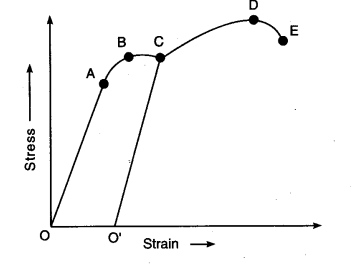# The stress-strain graph for a metal wire is given

#1

The stress-strain graph for a metal wire is given in figure, upto the point B, the wire returns to its original state O along the curve BAO, when it is gradually unloaded. Point E corresponds to the fracture point of the wire.(a)Upto which point of curve, is Hooke’s law obeyed ? This point is also called ‘Proportion limit’.
(b) Which point on the curve corresponds to elastic limit and yield point of the wire ?
© Indicate the elastic and plastic regions of the
stress-strain curve.
(d) What change happens when the wire is loaded upto a stress corresponding to point C on curve, and then unloaded gradually ?

#2

Ans. (a) Upto point A, Hooke’s law is obeyed because the graph is straight line from O to A.
(b) Point B, from the graph it is dear that the wire
returns to its original position after being unloaded upto point A only, henceJB is elastic limit.
© Elastic region—O to B
Plastic region—B to E
(d) Strain varies directly proportional to load upto
point A and after A, strain increases by greater amount as compared to first case (i.e., O to A) for a given increase in load. Beyond the elastic limit B, the curve does not retrace backwards as the wire is unloaded but returns along dotted line CO’. Point O’ corresponds to strain at zero load, which shows there is a permanent strain in the wire.

#3

What happens at D-E?